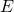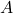# Understanding Variance Explained in PCA – Matrix Approximation

Principal component analysis (PCA from here on) is performed via linear algebra functions called eigen decomposition or singular value decomposition. Since you are actually reading this, you may well have used PCA in the past, at school or where you work. There is a strong link between PCA and the usual least squares regression (previous posts here and here). More recently I explained what does variance explained by the first principal component actually means.

This post offers a matrix approximation perspective. As a by-product, we also show how to compare two matrices, to see how different they are from each other. Matrix approximation is a bit math-hairy, but we keep it simple here I promise. For this fascinating field itself I suspect a rise in importance. We are constantly stretching what we can do computationally, and by using approximations rather than the actual data, we can ease that burden. The price for using approximation is decrease in accuracy (à la “garbage in garbage out”), but with good approximation the tradeoff between the accuracy and computational time is favorable.

If you apply the PCA to some matrix A, and you column-bind the first k principal components- call it matrix B, that B matrix is the best approximation for the original matrix A you can get. You get better and better approximation by increasing the k number, i.e. using more PCs, but utilizing only k columns you can’t have any better approximation.

Say you have 10 columns but you want to work with only 4, your k. The 4 principal components constitute the best algebraic approximation (again, which uses only 4 columns) to the original matrix. Change a single entry in that 4 column matrix, and you are moving away from your original A matrix. More details below, if you are interested to know a bit more about PCA internals.

Let’s expand the usual notion of distance between two points to matrices. Ifandare just numbers,is the euclidean distance between them. If they are vectors, sayis 5 numbers andis 5 numbers we compute the 5 quantitiesand sum them up. Matrices are no different, we sum up all the entries simply. We call this summation over rowsand columns:the Frobenius norm of a matrix E.could be thought of as the error matrix, the distance between the(the original) and your approximation. You can be happy with your approximation for A if the Frobenius norm of the errors between the entries A and B is small.

Coding wise you don’t need to program from scratch. Matrix::norm (R) and np.linalg.norm (Python) will do the trick.

### Illustration

The following code pulls some price data for 4 ETFs which will be ourmatrix, perform PCA and binds the first few principal components (AKA scores) which will be ourmatrix.

You can see that the approximation becomes better as k increases from 1 to 4. ret - mat_approx is our matrixin the math above. Using all 4 principal components we retrieve the original matrix:

The less principal components you use, the worse your approximation becomes (the norm of the E matrix becomes larger).

Coming back full circle to the start of the post where I mentioned that using PCA is a way to get the best approximation. Eckart and Young theorem tells us that the approximation we just made, is the best (mark: Frobenius norm speaking, for other norms it may not be the best), that we could have done. If you want to know more about this topic, it falls under the apt name of sketching. A good sketch matrix B is such that computations can be performed on B rather than on A without much loss in precision; sketching stands here for “not the actual picture but very economical and very clear”.

So on top of the statistical interpretation from previous PCA posts, you now also have this algebraic interpretation of PCA.